# Logical matrix

Jump to: navigation, search

A systemwhereis a non-empty set;;are binary operations; andis a unary operation on. Any formula of propositional logic, constructed from propositional variables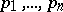by means of the logical connectives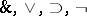, can be regarded as an-place function onifare assumed to be variables with range of valuesand the logical connectives are interpreted as the corresponding operations of the logical matrix. A formulais said to be generally valid in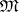if for any values of the variables inthe value ofbelongs to. A logical matrixis said to be characteristic for a propositional calculusif the formulas that are generally valid inare exactly those that are deducible in. An example of a logical matrix is the system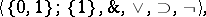where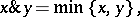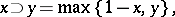This logical matrix is characteristic for the classical propositional calculus. t logic','../p/p110060.htm','Set theory','../s/s084750.htm','Syntax','../s/s091900.htm','Undecidability','../u/u095140.htm','Unsolvability','../u/u095800.htm','ZFC','../z/z130100.htm')" style="background-color:yellow;">K. Gödel proved that it is impossible to construct a logical matrix with a finite setthat is characteristic for the intuitionistic propositional calculus.